# NMath User's Guide

40.1 Distribution Classes (.NET, C#, CSharp, VB, Visual Basic, F#)

The NMath Stats probability distribution classes are listed in Table 27.

 Class Distribution Beta distribution Binomial distribution Chi-Square () distribution Exponential distribution F distribution Gamma distribution Geometric distribution JohnsonDistribution Johnson distribution Logistic distribution Log-normal distribution Negative Binomial distribution Normal (Gaussian) distribution Poisson distribution Student's t distribution Triangular distribution Uniform distribution Weibull distribution

All distribution classes share a common interface. Class is the abstract base class for the distribution classes, and provides the following abstract methods implemented by the derived classes:

PDF() computes the probability density function at a given x.

CDF() computes the cumulative distribution function at a given x.

InverseCDF() computes the inverse cumulative distribution function for a given probability p—that is, it returns x such that CDF( x ) = p.

In addition, all NMath Stats distribution classes implement the interface, which provides the following read-only properties:

Mean gets the mean of the distribution.

Variance gets the variance of the distribution.

Kurtosis gets the kurtosis of the distribution.

Skewness gets the skewness of the distribution.

Variance is the square of the standard deviation. Kurtosis is a measure of the degree of peakednesss of a distribution; skewness is a measure of the degree of asymmetry.

Once you have constructed a derived distribution type, you can query it for the PDF, CDF, inverse CDF, and random variable moments. For example, this code constructs a NormalDistribution with mean 0 and variance 1, then queries it:

Code Example – C# normal distribution

```var dist = new NormalDistribution( 0, 1 );
```
```double pdf = dist.PDF( 0 );
```
```double cdf = dist.CDF( 0 );
```
```double invCdf = dist.InverseCDF( .5 );
```
```double mean = dist.Mean;
```
```double var = dist.Variance;
```
```double kurt = dist.Kurtosis;
```
```double skew = dist.Skewness;
```

Code Example – VB normal distribution

```Dim Dist As New NormalDistribution(0, 1)
```
```Dim PDF As Double = Dist.PDF(0)
```
```Dim CDF As Double = Dist.CDF(0)
```
```Dim InvCDF As Double = Dist.InverseCDF(0.5)
```
```Dim Mean As Double = Dist.Mean
```
```Dim Var As Double = Dist.Variance
```
```Dim Kurt As Double = Dist.Kurtosis
```
```Dim Skew As Double = Dist.Skewness
```

Beta Distribution

Class represents the beta probability distribution. The beta distribution is a family of curves with two free parameters, usually labelled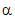and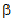. Beta distributions are nonzero only on the interval (0 1).

The distribution function for the beta distribution is:whereis the beta function. The beta CDF is the same as the incomplete beta function.

For example, this code constructs a BetaDistribution:

Code Example – C# beta distribution

```double alpha = 3;
```
```double beta = 7;
```
```var dist = new BetaDistribution( alpha, beta );
```

Code Example – VB beta distribution

```Dim Alpha As Double = 3
```
```Dim Beta As Double = 7
```
```Dim Dist As New BetaDistribution(Alpha, Beta)
```

The default constructor creates a BetaDistribution withand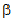equal to 1:

Code Example – C# beta distribution

```var dist = new BetaDistribution();
```

Code Example – VB beta distribution

```Dim Dist As New BetaDistribution()
```

The provided Alpha and Beta properties can be used to get and set the shape parameters after construction:

Code Example – C# beta distribution

```dist.Alpha = 4;
```
```dist.Beta = 10;
```

Code Example – VB beta distribution

```Dist.Alpha = 4
```
```Dist.Beta = 10
```

Once you have constructed a BetaDistribution object, you can query it for the PDF, CDF, inverse CDF, and random variable moments, as described in Section 40.1.

Binomial Distribution

Class represents the discrete probability distribution of obtaining exactly n successes in N trials where the probability of success on each trial is p. For example, this code constructs an BinomialDistribution:

Code Example – C# binomial distribution

```int n = 20;
```
```double p = 0.25;
```
```var bin = new BinomialDistribution( n, p );
```

Code Example – VB binomial distribution

```Dim N As Integer = 20
```
```Dim P As Double = 0.25
```
```Dim Bin As New BinomialDistribution(N, P)
```

The default constructor creates an BinomialDistribution with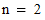and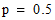:

Code Example – C# binomial distribution

```var bin = new BinomialDistribution();
```

Code Example – VB binomial distribution

```Dim Bin As New BinomialDistribution()
```

The provided N and P properties can be used to get and set the number of trials and the probability of success on each trial after construction:

Code Example – C# binomial distribution

```bin.N = 75;
```
```bin.P = 0.02;
```

Code Example – VB binomial distribution

```Bin.N = 75
```
```Bin.P = 0.02
```

Once you have constructed an BinomialDistribution object, you can query it for the PDF, CDF, inverse CDF, and random variable moments, as described in Section 40.1.

Chi-Square Distribution

Class represents the chi-square () probability distribution. The chi-square distribution is a special case of the gamma distribution with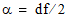and, where df is the degrees of freedom.

For example, this code constructs a ChiSquareDistribution:

Code Example – C# chi-square distribution

```double df = 16;
```
```var chiSq = new ChiSquareDistribution( df );
```

Code Example – VB chi-square distribution

```Dim DF As Double = 16
```
```Dim ChiSq As New ChiSquareDistribution(DF)
```

The default constructor creates a ChiSquareDistribution with 1 degree of freedom:

Code Example – C# chi-square distribution

```var chiSq = new ChiSquareDistribution();
```

Code Example – VB chi-square distribution

```Dim ChiSq As New ChiSquareDistribution()
```

The provided DegreesOfFreedom property can be used to get and set the degrees of freedom of the distribution after construction:

Code Example – C# chi-square distribution

```chiSq.DegreesOfFreedom = 10;
```

Code Example – VB chi-square distribution

```ChiSq.DegreesOfFreedom = 10
```

Once you have constructed a ChiSquareDistribution object, you can query it for the PDF, CDF, inverse CDF, and random variable moments, as described in Section 40.1.

Exponential Distribution

Class represents the exponential distribution. A random variable w is said to have an exponential distribution if it has a probability density function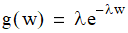where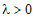is often called the rate parameter. The mean of an exponential distribution is, and the variance is. For example, this code constructs an ExponentialDistribution:

Code Example – C# exponential distribution

```double lambda = 22;
```
```var exp = new ExponentialDistribution( lambda );
```

Code Example – VB exponential distribution

```Dim Lambda As Double = 22
```
```Dim Exp As New ExponentialDistribution(Lambda)
```

The provided Lambda property can be used to get and set the rate after construction:

Code Example – C# exponential distribution

```exp.Lambda = 15;
```

Code Example – VB exponential distribution

```Exp.Lambda = 15
```

Once you have constructed an ExponentialDistribution object, you can query it for the PDF, CDF, inverse CDF, and random variable moments, as described in Section 40.1.

F Distribution

Class represents the F probability distribution. The F distribution is the ratio of two chi-square distributions with degrees of freedom df1 and df2, respectively, where each chi-square has first been divided by its degrees of freedom. For example, this code constructs an FDistribution:

Code Example – C# F distribution

```double df1 = 11;
```
```double df2 = 19;
```
```var f = new FDistribution( df1, df2 );
```

Code Example – VB F distribution

```Dim DF1 As Double = 11
```
```Dim DF2 As Double = 19
```
```Dim F As New FDistribution(DF1, DF2)
```

The default constructor creates an FDistribution with both degrees of freedom equal to 1:

Code Example – C# F distribution

```var f = new FDistribution();
```

Code Example – VB F distribution

```Dim F As New FDistribution()
```

The provided DegreesOfFreedom1 and DegreesOfFreedom2 properties can be used to get and set the degrees of freedom after construction:

Code Example – C# F distribution

```f.DegreesOfFreedom1 = 15;
```
```f.DegreesOfFreedom2 = 23;
```

Code Example – VB F distribution

```F.DegreesOfFreedom1 = 15
```
```F.DegreesOfFreedom2 = 23
```

Once you have constructed an FDistribution object, you can query it for the PDF, CDF, inverse CDF, and random variable moments, as described in Section 40.1.

Gamma Distribution

Class represents the gamma probability distribution. The gamma distribution is a family of curves with two free parameters, usually labelledand. The mean of the distribution is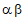; the variance is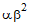. Whenis large, the gamma distribution closely approximates a normal distribution.

The distribution function for the gamma distribution is: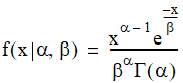where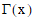is the Gamma function.

For example, this code constructs a GammaDistribution:

Code Example – C# gamma distribution

```double alpha = 7;
```
```double beta = 12;
```
```var gamma = new GammaDistribution( alpha, beta );
```

Code Example – VB gamma distribution

```Dim Alpha As Double = 7
```
```Dim Beta As Double = 12
```
```Dim Gamma As New GammaDistribution(Alpha, Beta)
```

The default constructor creates a GammaDistribution withandequal to 1:

Code Example – C# gamma distribution

```var gamma = new GammaDistribution();
```

Code Example – VB gamma distribution

```Dim Gamma As New GammaDistribution()
```

The provided Alpha and Beta properties can be used to get and set the shape parameters after construction:

Code Example – C# gamma distribution

```gamma.Alpha = 10;
```
```gamma.Beta = 15;
```

Code Example – VB gamma distribution

```Gamma.Alpha = 10
```
```Gamma.Beta = 15
```

Once you have constructed a GammaDistribution object, you can query it for the PDF, CDF, inverse CDF, and random variable moments, as described in Section 40.1.

Geometric Distribution

Class represents the geometric distribution. The geometric distribution is the probability distribution of the number of failures before the first success. It is supported on the set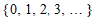.

A GeometricDistribution is constructed from a given probability of success p, where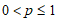. For example:

Code Example – C# geometric distribution

```double p = .25;
```
```var geo = new GeometricDistribution( p );
```

Code Example – VB geometric distribution

```Dim P As Double = 0.25
```
```Dim Geo As New GeometricDistribution(P)
```

Class GeometricDistribution provides property P that gets and sets the probability for success for the distribution.

Code Example – C# geometric distribution

```geo.P = .5;
```

Code Example – VB geometric distribution

```Geo.P = 0.5
```

Once you have constructed a GeometricDistribution object, you can query it for the PDF, CDF, inverse CDF, and random variable moments, as described in Section 40.1.

Johnson Distribution

Class represents the Johnson system of distributions. The Johnson system is based on three possible transformations of a normal random variable—exponential, logistic, and hyperbolic sine—plus the identity transformation:where the transformation f() has four possible forms based on the distribution type:

Normal (SN): f(u) = exp(u)

Log Normal (SL): f(u) = u

Unbounded (SU): f(u) = u + sqrt(1+u^2)

Bounded (SB): f(u) = u/(1-u)

A JohnsonDistribution instance is constructed from a set of distribution parameter values, and a JohnsonTransformationType enumerated value specifying the transformation type. For instance:

Code Example – C# Johnson distribution

```double gamma = -0.18;
```
```double delta = 2.55;
```
```double xi = -0.14;
```
```double lambda = 2.35;
```
```JohnsonTransformationType type = JohnsonTransformationType.Normal;

```

```var dist =
```
```  new JohnsonDistribution( gamma, delta, xi, lambda, type );
```

Code Example – VB Johnson distribution

```Dim Gamma As Double = -0.18
```
```Dim Delta As Double = 2.55
```
```Dim Xi As Double = -0.14
```
```Dim Lambda As Double = 2.35
```
```Dim Type As JohnsonTransformationType =
```
```  JohnsonTransformationType.Normal

```

```Dim Dist As New JohnsonDistribution(Gamma, Delta, Xi, Lambda, Type)
```

Once you have constructed a JohnsonDistribution object, you can query it for the PDF, CDF, inverse CDF, and random variable moments, as described in Section 40.1.

Class JohnsonDistribution also provides a static Fit() method for fitting a Johnson distribution to a data set. Estimation of the Johnson parameters is done from quantiles that correspond to the cumulative probabilities [0.05, 0.206, 0.5, 0.794, 0.95] using the method of Wheeler (1980).1 For example:

Code Example – C# Johnson distribution

```var data = new DoubleVector(-0.09736927, 0.21615254,
```
```  0.88246516, 0.20559750, -0.61643584, -0.73479925, -0.13180279,
```
```  0.31001699, -1.03968035, -0.18430887, 0.96726726, -0.10828009, -
```
```  0.69842067, -0.27594517, 1.11464855, 0.55004396, 1.23667580,
```
```  0.13909786, 0.41027510, -0.55845691);
```
```var dist = JohnsonDistribution.Fit(data);
```

Code Example – VB Johnson distribution

```Dim Data As New DoubleVector(-0.09736927, 0.21615254,
```
```  0.88246516, 0.2055975, -0.61643584, -0.73479925, -0.13180279,
```
```  0.31001699, -1.03968035, -0.18430887, 0.96726726, -0.10828009,
```
```  -0.69842067, -0.27594517, 1.11464855, 0.55004396, 1.2366758,
```
```  0.13909786, 0.4102751, -0.55845691)
```
```Dim Dist As JohnsonDistribution = JohnsonDistribution.Fit(Data)
```

The Transform() method transforms data using a JohnsonDistribution object.

Logistic Distribution

Class represents the logistic probability distribution with a specified location (mean) and scale. The logistic distribution with location m and scale b has distribution function: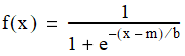and density: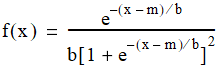For example, this code constructs a :

Code Example – C# logistic distribution

```double loc = 2.0;
```
```double scale = 1.5;
```
```var logistic = new LogisticDistribution( loc, scale );
```

Code Example – VB logistic distribution

```Dim Loc As Double = 2.0
```
```Dim Scale As Double = 1.5
```
```Dim Logistic As New LogisticDistribution(Loc, Scale)
```

The provided Location and Scale properties can be used to get and set distribution parameters after construction:

Code Example – C# logistic distribution

```logistic.Location = 7.123;
```
```logistic.Scale = 4.5;
```

Code Example – VB logistic distribution

```Logistic.Location = 7.123
```
```Logistic.Scale = 4.5
```

Once you have constructed a object, you can query it for the PDF, CDF, inverse CDF, and random variable moments, as described in Section 40.1.

Log-Normal Distribution

Class represents the log-normal distribution. A log-normal distribution has a normal distribution as its logarithm: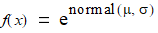For example, this code constructs an LognormalDistribution whose associated normal distribution has the specified mean and standard deviation:

Code Example – C# log-normal distribution

```double mu = -99;
```
```double sigma = 6;
```
```var ln = new LognormalDistribution( mu, sigma );
```

Code Example – VB log-normal distribution

```Dim Mu As Double = -99
```
```Dim Sigma As Double = 6
```
```Dim LN As New LognormalDistribution(Mu, Sigma)
```

The default constructor creates a LognormalDistribution whose associated normal distribution has mean 0 and standard deviation 1:

Code Example – C# log-normal distribution

```var ln = new LognormalDistribution();
```

Code Example – VB log-normal distribution

```Dim LN As New LognormalDistribution()
```

The Mu and Sigma properties can be used to get and set the mean and standard deviation after construction:

Code Example – C# log-normal distribution

```ln.Mu = 2.25;
```
```ln.Sigma = .75;
```

Code Example – VB log-normal distribution

```LN.Mu = 2.25
```
```LN.Sigma = 0.75
```

Once you have constructed a LognormalDistribution object, you can query it for the PDF, CDF, inverse CDF, and random variable moments, as described in Section 40.1.

Negative Binomial Distribution

Class represents the discrete probability distribution of obtaining N successes in a series of x trials, where the probability of success on each trial is P.

For example, this code constructs an NegativeBinomialDistribution:

Code Example – C# negative binomial distribution

```int n = 5;
```
```double p = 0.25;
```
```var negBin = new NegativeBinomialDistribution( n, p );
```

Code Example – VB negative binomial distribution

```Dim N As Integer = 5
```
```Dim P As Double = 0.25
```
```Dim NegBin As New NegativeBinomialDistribution(N, P)
```

The default constructor creates an NegativeBinomialDistribution with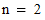and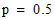:

Code Example – C# negative binomial distribution

```var negBin = new NegativeBinomialDistribution();
```

Code Example – VB negative binomial distribution

```Dim NegBin As New NegativeBinomialDistribution()
```

The provided N and P properties can be used to get and set the number of successes and the probability of success on each trial after construction:

Code Example – C# negative binomial distribution

```negBin.N = 75;
```
```negBin.P = 0.02;
```

Code Example – VB negative binomial distribution

```Bin.N = 75
```
```Bin.P = 0.02
```

Once you have constructed an NegativeBinomialDistribution object, you can query it for the PDF, CDF, inverse CDF, and random variable moments, as described in Section 40.1.

Normal Distribution

Class represents the normal (Gaussian) probability distribution. with a specified mean and variance. For example, this code creates a normal distribution with a mean of 1 and variance of 2.5:

Code Example – C# normal distribution

```var norm = new NormalDistribution( 1, 2.5 );
```

Code Example – VB normal distribution

```Dim Norm As New NormalDistribution(1, 2.5)
```

The default constructor creates a NormalDistribution with mean 0 and variance 1:

Code Example – C# normal distribution

```var norm = new NormalDistribution();
```

Code Example – VB normal distribution

```Dim Norm As New NormalDistribution()
```

The Mean and Variance properties inherited from IRandomVariableMoments can be used to get and set the mean and variance after construction:

Code Example – C# normal distribution

```norm.Mean = 2.25;
```
```norm.Variance = .75;
```

Code Example – VB normal distribution

```Norm.Mean = 2.25
```
```Norm.Variance = 0.75
```

Once you have constructed a NormalDistribution object, you can query it for the PDF, CDF, inverse CDF, and random variable moments, as described in Section 40.1.

Poisson Distribution

Class represents a poisson distribution with a specifiedparameter, which is both the mean and the variance of the distribution. The poisson distribution is the probability of obtaining exactly n successes in N trials. It is often used as a model for the number of events in a specific time period. Poisson (1837) showed that the Poisson distribution is the limiting case of a binomial distribution where N approaches infinity and p goes to zero while. The distribution function for the Poisson distribution is: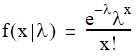For example, this code constructs a PoissonDistribution:

Code Example – C# poisson distribution

```double lambda = 150;
```
```var poisson = new PoissonDistribution( lambda );
```

Code Example – VB poisson distribution

```Dim Lambda As Double = 150
```
```Dim Poisson As New PoissonDistribution(Lambda)
```

The Mean and Variance properties inherited from IRandomVariableMoments can also be used to get and set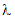after construction:

Code Example – C# poisson distribution

```poisson.Mean = 3;
```

Code Example – VB poisson distribution

```Poisson.Mean = 3
```

Once you have constructed a PoissonDistribution object, you can query it for the PDF, CDF, inverse CDF, and random variable moments, as described in Section 40.1.

Student's t Distribution

Class represents Student's t distribution with specified degrees of freedom. As the number of degrees of freedom grows, the t distribution approaches the normal distribution with mean 0 and variance 1.

For example, this code constructs a TDistribution:

Code Example – C# t distribution

```double df = 53;
```
```var t = new TDistribution( df );
```

Code Example – VB t distribution

```Dim DF As Double = 53
```
```Dim T As New TDistribution(DF)
```

The default constructor creates a TDistribution with 1 degree of freedom:

Code Example – C# t distribution

```var t = new TDistribution();
```

Code Example – VB t distribution

```Dim T As New TDistribution()
```

The provided DegreesOfFreedom property can be used to get and set the degrees of freedom of the distribution after construction:

Code Example – C# t distribution

```t.DegreesOfFreedom = 54;
```

Code Example – VB t distribution

```T.DegreesOfFreedom = 54
```

Once you have constructed a TDistribution object, you can query it for the PDF, CDF, inverse CDF, and random variable moments, as described in Section 40.1.

Triangular Distribution

Class represents the triangular distribution. The triangular distribution is defined by three parameters, a lower limit a, an upper limit b, and number c, between a and b, called the mode. The probability density function has the shape of a triangle in the X/Y plane with vertices (a, 0), (b, 0), and (c, y), where y is chosen so that the area of the triangle is 1.

For example, this code constructs an TriangularDistribution with the given parameters:

Code Example – C# triangular distribution

```double lower = 3;
```
```double upper = 10;
```
```double mode = 8;
```
```var td = new TriangularDistribution( lower, upper, mode );
```

Code Example – VB triangular distribution

```Dim Lower As Double = 3
```
```Dim Upper As Double = 10
```
```Dim Mode As Double = 8
```
```Dim TD As New TriangularDistribution(Lower, Upper, Mode)
```

If you don't specify the mode, the midpoint of the lower and upper limits is used.

The default constructor creates a TriangularDistribution with lower limit 0, upper limit 1, and mode 0.5:

Code Example – C# triangular distribution

```var td = new TriangularDistribution();
```

Code Example – VB triangular distribution

```Dim TD As New TriangularDistribution()
```

The LowerLimit, UpperLimit, and Mode properties can be used to get and set the distribution parameters after construction:

Code Example – C# triangular distribution

```td.LowerLimit = 1.5;
```
```td.UpperLimit = 3.5;
```
```td.Mode = 2.75;
```

Code Example – VB triangular distribution

```TD.LowerLimit = 1.5
```
```TD.UpperLimit = 3.5
```
```TD.Mode = 2.75
```

Once you have constructed a TriangularDistribution object, you can query it for the PDF, CDF, inverse CDF, and random variable moments, as described in Section 40.1.

Uniform Distribution

Class represents the uniform distribution. For example, this code constructs an UniformDistribution with the specified lower and upper limits:

Code Example – C# uniform distribution

```double lower = -.77;
```
```double upper = 1.22;
```
```var uni = new UniformDistribution( lower, upper );
```

Code Example – VB uniform distribution

```Dim Lower As Double = -0.77
```
```Dim Upper As Double = 1.22
```
```Dim Uni As New UniformDistribution(Lower, Upper)
```

The default constructor creates a UniformDistribution with lower limit 0 and upper limit 1:

Code Example – C# uniform distribution

```var uni = new UniformDistribution();
```

Code Example – VB uniform distribution

```Dim Uni As New UniformDistribution()
```

The LowerLimit and UpperLimit properties can be used to get and set the lower and upper limits after construction:

Code Example – C# uniform distribution

```uni.LowerLimit = 0;
```
```uni.UpperLimit = 2.0;
```

Code Example – VB uniform distribution

```Uni.LowerLimit = 0
```
```Uni.UpperLimit = 2.0
```

Once you have constructed a UniformDistribution object, you can query it for the PDF, CDF, inverse CDF, and random variable moments, as described in Section 40.1.

Weibull Distribution

Class represents the Weibull distribution. The probability density function of the Weibull distribution is given by: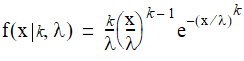where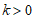is the shape parameter andis the scale parameter of the distribution.

For example, this code constructs an WeibullDistribution with the specified distribution parameters:

Code Example – C# Weibull distribution

```double scale = 1.5;
```
```double shape = 3;
```
```var wb = new WeibullDistribution( scale, shape );
```

Code Example – VB Weibull distribution

```Dim Scale As Double = 1.5
```
```Dim Shape As Double = 3
```
```Dim WB As New WeibullDistribution(Scale, Shape)
```

The Scale and Shape properties can be used to get and set the distribution parameters after construction:

Code Example – C# Weibull distribution

```wb.Scale = .5;
```
```wb.Shape = 2;
```

Code Example – VB Weibull distribution

```WB.Scale = 0.5
```
```WB.Shape = 2
```

Once you have constructed a WeibullDistribution object, you can query it for the PDF, CDF, inverse CDF, and random variable moments, as described in Section 40.1.

1. Wheeler, R.E. (1980). Quantile estimators of Johnson curve parameters. Biometrika. 67-3 725-728.

Top

Top